# 8.6. Decoding a Datatype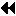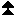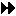Up: External Interfaces Next: MPI and Threads Previous: Error Classes, Error Codes, and Error Handlers

MPI-1 provides datatype objects, which allow users to specify an arbitrary layout of data in memory. The layout information, once put in a datatype, could not be decoded from the datatype. There are several cases, however, where accessing the layout information in opaque datatype objects would be useful.

The two functions in this section are used together to decode datatypes to recreate the calling sequence used in their initial definition. These can be used to allow a user to determine the type map and type signature of a datatype.

[ IN datatype] datatype to access (handle)
[ OUT num_integers] number of input integers used in the call constructing combiner (nonnegative integer)
[ OUT num_addresses] number of input addresses used in the call constructing combiner (nonnegative integer)
[ OUT num_datatypes] number of input datatypes used in the call constructing combiner (nonnegative integer)
[ OUT combiner] combiner (state)

int MPI_Type_get_envelope(MPI_Datatype datatype, int *num_integers, int *num_addresses, int *num_datatypes, int *combiner)

MPI_TYPE_GET_ENVELOPE(DATATYPE, NUM_INTEGERS, NUM_ADDRESSES, NUM_DATATYPES, COMBINER, IERROR)
INTEGER DATATYPE, NUM_INTEGERS, NUM_ADDRESSES, NUM_DATATYPES, COMBINER, IERROR

void MPI::Datatype::Get_envelope(int& num_integers, int& num_addresses, int& num_datatypes, int& combiner) const

For the given datatype, MPI_TYPE_GET_ENVELOPE returns information on the number and type of input arguments used in the call that created the datatype. The number-of-arguments values returned can be used to provide sufficiently large arrays in the decoding routine MPI_TYPE_GET_CONTENTS. This call and the meaning of the returned values is described below. The combiner reflects the MPI datatype constructor call that was used in creating datatype.

[] Rationale.

By requiring that the combiner reflect the constructor used in the creation of the datatype, the decoded information can be used to effectively recreate the calling sequence used in the original creation. One call is effectively the same as another when the information obtained from MPI_TYPE_GET_CONTENTS may be used with either to produce the same outcome. C calls MPI_Type_hindexed and MPI_Type_create_hindexed are always effectively the same while the Fortran call MPI_TYPE_HINDEXED will be different than either of these in some MPI implementations. This is the most useful information and was felt to be reasonable even though it constrains implementations to remember the original constructor sequence even if the internal representation is different.

The decoded information keeps track of datatype duplications. This is important as one needs to distinguish between a predefined datatype and a dup of a predefined datatype. The former is a constant object that cannot be freed, while the latter is a derived datatype that can be freed. ( End of rationale.)
The list below has the values that can be returned in combiner on the left and the call associated with them on the right.

[ MPI_COMBINER_NAMED]a named predefined datatype
[ MPI_COMBINER_DUP] MPI_TYPE_DUP
[ MPI_COMBINER_CONTIGUOUS] MPI_TYPE_CONTIGUOUS
[ MPI_COMBINER_VECTOR] MPI_TYPE_VECTOR
[ MPI_COMBINER_HVECTOR_INTEGER] MPI_TYPE_HVECTOR from Fortran
[ MPI_COMBINER_HVECTOR] MPI_TYPE_HVECTOR from C or C++
[ ] and in some case Fortran
[ ] or MPI_TYPE_CREATE_HVECTOR
[ MPI_COMBINER_INDEXED] MPI_TYPE_INDEXED
[ MPI_COMBINER_HINDEXED_INTEGER] MPI_TYPE_HINDEXED from Fortran
[ MPI_COMBINER_HINDEXED] MPI_TYPE_HINDEXED from C or C++
[ ] and in some case Fortran
[ ] or MPI_TYPE_CREATE_HINDEXED
[ MPI_COMBINER_INDEXED_BLOCK] MPI_TYPE_CREATE_INDEXED_BLOCK
[ MPI_COMBINER_STRUCT_INTEGER] MPI_TYPE_STRUCT from Fortran
[ MPI_COMBINER_STRUCT] MPI_TYPE_STRUCT from C or C++
[ ] and in some case Fortran
[ ] or MPI_TYPE_CREATE_STRUCT
[ MPI_COMBINER_SUBARRAY] MPI_TYPE_CREATE_SUBARRAY
[ MPI_COMBINER_DARRAY] MPI_TYPE_CREATE_DARRAY
[ MPI_COMBINER_F90_REAL] MPI_TYPE_CREATE_F90_REAL
[ MPI_COMBINER_F90_COMPLEX] MPI_TYPE_CREATE_F90_COMPLEX
[ MPI_COMBINER_F90_INTEGER] MPI_TYPE_CREATE_F90_INTEGER
[ MPI_COMBINER_RESIZED] MPI_TYPE_CREATE_RESIZED

If combiner is MPI_COMBINER_NAMED then datatype is a named predefined datatype.

For calls with address arguments, we sometimes need to differentiate whether the call used an integer or an address size argument. For example, there are two combiners for hvector: MPI_COMBINER_HVECTOR_INTEGER and MPI_COMBINER_HVECTOR. The former is used if it was the MPI-1 call from Fortran, and the latter is used if it was the MPI-1 call from C or C++. However, on systems where MPI_ADDRESS_KIND = MPI_INTEGER_KIND (i.e., where integer arguments and address size arguments are the same), the combiner MPI_COMBINER_HVECTOR may be returned for a datatype constructed by a call to MPI_TYPE_HVECTOR from Fortran. Similarly, MPI_COMBINER_HINDEXED may be returned for a datatype constructed by a call to MPI_TYPE_HINDEXED from Fortran, and MPI_COMBINER_STRUCT may be returned for a datatype constructed by a call to MPI_TYPE_STRUCT from Fortran. On such systems, one need not differentiate constructors that take address size arguments from constructors that take integer arguments, since these are the same. The new MPI-2 calls all use address sized arguments.

[] Rationale.

For recreating the original call, it is important to know if address information may have been truncated. The MPI-1 calls from Fortran for a few routines could be subject to truncation in the case where the default INTEGER size is smaller than the size of an address. ( End of rationale.)
The actual arguments used in the creation call for a datatype can be obtained from the call:

[ IN datatype] datatype to access (handle)
[ IN max_integers] number of elements in array_of_integers (non-negative integer)
[ IN max_datatypes] number of elements in array_of_datatypes (non-negative integer)
[ OUT array_of_integers] contains integer arguments used in constructing datatype (array of integers)
[ OUT array_of_addresses] contains address arguments used in constructing datatype (array of integers)
[ OUT array_of_datatypes] contains datatype arguments used in constructing datatype (array of handles)

int MPI_Type_get_contents(MPI_Datatype datatype, int max_integers, int max_addresses, int max_datatypes, int array_of_integers[], MPI_Aint array_of_addresses[], MPI_Datatype array_of_datatypes[])

INTEGER DATATYPE, MAX_INTEGERS, MAX_ADDRESSES, MAX_DATATYPES, ARRAY_OF_INTEGERS(*), ARRAY_OF_DATATYPES(*), IERROR

void MPI::Datatype::Get_contents(int max_integers, int max_addresses, int max_datatypes, int array_of_integers[], MPI::Aint array_of_addresses[], MPI::Datatype array_of_datatypes[]) const

datatype must be a predefined unnamed or a derived datatype; the call is erroneous if datatype is a predefined named datatype.

The values given for max_integers, max_addresses, and max_datatypes must be at least as large as the value returned in num_integers, num_addresses, and num_datatypes, respectively, in the call MPI_TYPE_GET_ENVELOPE for the same datatype argument.

[] Rationale.

The arguments max_integers, max_addresses, and max_datatypes allow for error checking in the call. This is analogous to the topology calls in MPI-1. ( End of rationale.)
The datatypes returned in array_of_datatypes are handles to datatype objects that are equivalent to the datatypes used in the original construction call. If these were derived datatypes, then the returned datatypes are new datatype objects, and the user is responsible for freeing these datatypes with MPI_TYPE_FREE. If these were predefined datatypes, then the returned datatype is equal to that (constant) predefined datatype and cannot be freed.

The committed state of returned derived datatypes is undefined, i.e., the datatypes may or may not be committed. Furthermore, the content of attributes of returned datatypes is undefined.

Note that MPI_TYPE_GET_CONTENTS can be invoked with a datatype argument that was constructed using MPI_TYPE_CREATE_F90_REAL, MPI_TYPE_CREATE_F90_INTEGER, or MPI_TYPE_CREATE_F90_COMPLEX (an unnamed predefined datatype). In such a case, an empty array_of_datatypes is returned.

[] Rationale.

The definition of datatype equivalence implies that equivalent predefined datatypes are equal. By requiring the same handle for named predefined datatypes, it is possible to use the == or .EQ. comparison operator to determine the datatype involved. ( End of rationale.)

The datatypes returned in array_of_datatypes must appear to the user as if each is an equivalent copy of the datatype used in the type constructor call. Whether this is done by creating a new datatype or via another mechanism such as a reference count mechanism is up to the implementation as long as the semantics are preserved. ( End of advice to implementors.)

[] Rationale.

The committed state and attributes of the returned datatype is deliberately left vague. The datatype used in the original construction may have been modified since its use in the constructor call. Attributes can be added, removed, or modified as well as having the datatype committed. The semantics given allow for a reference count implementation without having to track these changes. ( End of rationale.)

In the MPI-1 datatype constructor calls, the address arguments in Fortran are of type INTEGER. In the new MPI-2 calls, the address arguments are of type INTEGER(KIND=MPI_ADDRESS_KIND). The call MPI_TYPE_GET_CONTENTS returns all addresses in an argument of type INTEGER(KIND=MPI_ADDRESS_KIND). This is true even if the old MPI-1 calls were used. Thus, the location of values returned can be thought of as being returned by the C bindings. It can also be determined by examining the new MPI-2 calls for datatype constructors for the deprecated MPI-1 calls that involve addresses.

[] Rationale.

By having all address arguments returned in the array_of_addresses argument, the result from a C and Fortran decoding of a datatype gives the result in the same argument. It is assumed that an integer of type INTEGER(KIND=MPI_ADDRESS_KIND) will be at least as large as the INTEGER argument used in datatype construction with the old MPI-1 calls so no loss of information will occur. ( End of rationale.)
The following defines what values are placed in each entry of the returned arrays depending on the datatype constructor used for datatype. It also specifies the size of the arrays needed which is the values returned by MPI_TYPE_GET_ENVELOPE. In Fortran, the following calls were made:

```PARAMETER (LARGE = 1000)
INTEGER TYPE, NI, NA, ND, COMBINER, I(LARGE), D(LARGE), IERROR
!     CONSTRUCT DATATYPE TYPE (NOT SHOWN)
CALL MPI_TYPE_GET_ENVELOPE(TYPE, NI, NA, ND, COMBINER, IERROR)
IF ((NI .GT. LARGE) .OR. (NA .GT. LARGE) .OR. (ND .GT. LARGE)) THEN
WRITE (*, *) "NI, NA, OR ND = ", NI, NA, ND, &
" RETURNED BY MPI_TYPE_GET_ENVELOPE IS LARGER THAN LARGE = ", LARGE
CALL MPI_ABORT(MPI_COMM_WORLD, 99)
ENDIF
CALL MPI_TYPE_GET_CONTENTS(TYPE, NI, NA, ND, I, A, D, IERROR)
```
or in C the analogous calls of:

```#define LARGE 1000
int ni, na, nd, combiner, i[LARGE];
MPI_Aint a[LARGE];
MPI_Datatype type, d[LARGE];
/* construct datatype type (not shown) */
MPI_Type_get_envelope(type, &ni, &na, &nd, &combiner);
if ((ni > LARGE) || (na > LARGE) || (nd > LARGE)) {
fprintf(stderr, "ni, na, or nd = %d %d %d returned by ", ni, na, nd);
fprintf(stderr, "MPI_Type_get_envelope is larger than LARGE = %d\n",
LARGE);
MPI_Abort(MPI_COMM_WORLD, 99);
};
MPI_Type_get_contents(type, ni, na, nd, i, a, d);
```
The C++ code is in analogy to the C code above with the same values returned.

In the descriptions that follow, the lower case name of arguments is used.

If combiner is MPI_COMBINER_NAMED then it is erroneous to call MPI_TYPE_GET_CONTENTS.

If combiner is MPI_COMBINER_DUP then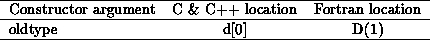and ni = 0, na = 0, nd = 1.

If combiner is MPI_COMBINER_CONTIGUOUS then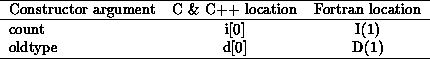and ni = 1, na = 0, nd = 1.

If combiner is MPI_COMBINER_VECTOR then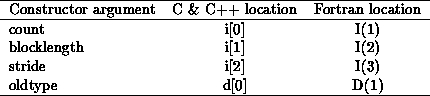and ni = 3, na = 0, nd = 1.

If combiner is MPI_COMBINER_HVECTOR_INTEGER or MPI_COMBINER_HVECTOR then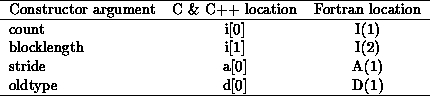and ni = 2, na = 1, nd = 1.

If combiner is MPI_COMBINER_INDEXED then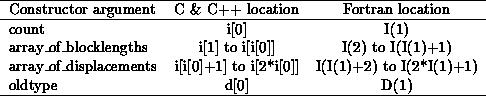and ni = 2*count+1, na = 0, nd = 1.

If combiner is MPI_COMBINER_HINDEXED_INTEGER or MPI_COMBINER_HINDEXED thenand ni = count+1, na = count, nd = 1.

If combiner is MPI_COMBINER_INDEXED_BLOCK then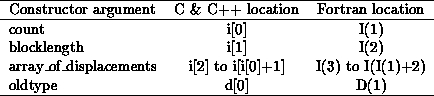and ni = count+2, na = 0, nd = 1.

If combiner is MPI_COMBINER_STRUCT_INTEGER or MPI_COMBINER_STRUCT then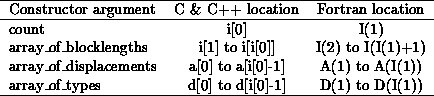and ni = count+1, na = count, nd = count.

If combiner is MPI_COMBINER_SUBARRAY then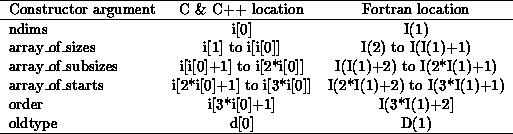and ni = 3*ndims+2, na = 0, nd = 1.

If combiner is MPI_COMBINER_DARRAY then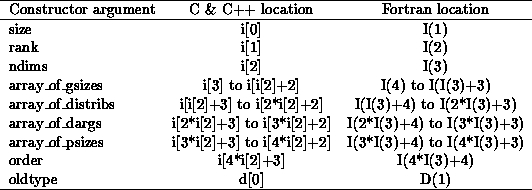and ni = 4*ndims+4, na = 0, nd = 1.

If combiner is MPI_COMBINER_F90_REAL then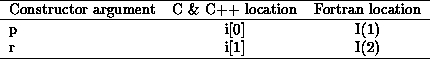and ni = 2, na = 0, nd = 0.

If combiner is MPI_COMBINER_F90_COMPLEX thenand ni = 2, na = 0, nd = 0.

If combiner is MPI_COMBINER_F90_INTEGER then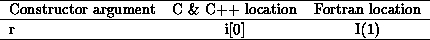and ni = 1, na = 0, nd = 0.

If combiner is MPI_COMBINER_RESIZED then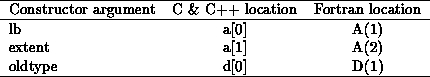and ni = 0, na = 2, nd = 1.

Example This example shows how a datatype can be decoded. The routine printdatatype prints out the elements of the datatype. Note the use of MPI_Type_free for datatypes that are not predefined.

```/*
Example of decoding a datatype.

Returns 0 if the datatype is predefined, 1 otherwise
*/
#include <stdio.h>
#include <stdlib.h>
#include "mpi.h"
int printdatatype( MPI_Datatype datatype )
{
int *array_of_ints;
MPI_Datatype *array_of_dtypes;
int i;

MPI_Type_get_envelope( datatype,
switch (combiner) {
case MPI_COMBINER_NAMED:
printf( "Datatype is named:" );
/* To print the specific type, we can match against the
predefined forms. We can NOT use a switch statement here
We could also use MPI_TYPE_GET_NAME if we prefered to use
names that the user may have changed.
*/
if      (datatype == MPI_INT)    printf( "MPI_INT\n" );
else if (datatype == MPI_DOUBLE) printf( "MPI_DOUBLE\n" );
... else test for other types ...
return 0;
break;
case MPI_COMBINER_STRUCT:
case MPI_COMBINER_STRUCT_INTEGER:
printf( "Datatype is struct containing" );
array_of_ints   = (int *)malloc( num_ints * sizeof(int) );
(MPI_Aint *) malloc( num_adds * sizeof(MPI_Aint) );
array_of_dtypes = (MPI_Datatype *)
malloc( num_dtypes * sizeof(MPI_Datatype) );
printf( " %d datatypes:\n", array_of_ints );
for (i=0; i<array_of_ints; i++) {
printf( "blocklength %d, displacement %ld, type:\n",
if (printdatatype( array_of_dtypes[i] )) {
/* Note that we free the type ONLY if it
is not predefined */
MPI_Type_free( &array_of_dtypes[i] );
}
}
free( array_of_ints );
free( array_of_dtypes );
break;
... other combiner values ...
default:
printf( "Unrecognized combiner type\n" );
}
return 1;
}
```Up: External Interfaces Next: MPI and Threads Previous: Error Classes, Error Codes, and Error Handlers